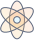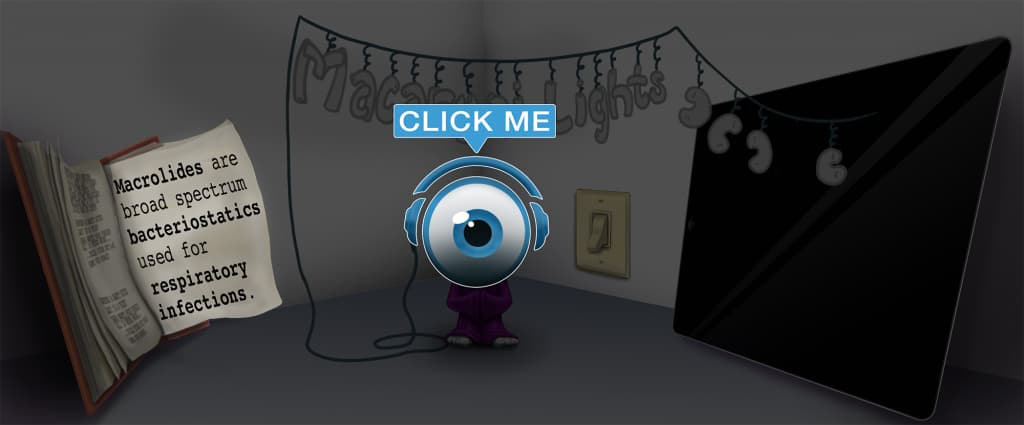# Master Conversions: Volume with Picmonic for Nursing RN

## With Picmonic, facts become pictures. We've taken what the science shows - image mnemonics work - but we've boosted the effectiveness by building and associating memorable characters, interesting audio stories, and built-in quizzing.

### Conversions: Volume

Volume-cupPicmonic
Conversion is the first step in dosage calculations for medication administration, as medications may not always be prescribed in the same unit. Memorizing the conversions will help you with dosage calculations and administration. For volume, one milliliter (mL) is equivalent to one cubic centimeter (cc). Five milliliters (mL) is equivalent to one teaspoon (tsp). Fifteen milliliters (mL) is equivalent to one tablespoon (tbsp). Thirty milliliters (mL) is equivalent to one fluid ounce (oz). One thousand milliliters (mL) is equivalent to one liter (L). Two tablespoons (tbsp) equivalent to one fluid ounce (oz). Eight fluid ounces (oz) is equivalent to one cup.
7 KEY FACTS
VOLUME
1 mL = 1 cc
(1) Wand Millionaire-liter-of-cola = 1 Cubic-cat

One milliliter (mL) is equivalent to 1 cc (cubic centimeter).

5 mL = 1 Tsp
(5) Hand Millionaire-liter-of-cola = (1) Wand Tea-spoon

Five milliliters (mL) is equivalent to 1 teaspoon (tsp). To convert teaspoons to milliliters, multiply the number of teaspoons by 5.

15 mL = 1 Tbsp
(15) Quinceañera Millionaire-liter-of-cola + (1) Wand Table-spoon

Fifteen milliliters (mL) is equivalent to 1 tablespoons (tbsp). To convert tablespoons to milliliters, multiply the number of tablespoons by 15.

30 mL = 1 oz
(30) Dirty Millionaire-liter-of-cola = (1) Wand Wizard-of-oz

Thirty milliliters (mL) is equivalent to 1 fluid ounce (oz). To convert ounces to milliliters, multiply the number of ounces by 30.

1000 mL = 1L
1 Thou(SAND) Millionaire-liter-of-cola = (1) Wand Liter-of-cola

One thousand milliliters (mL) is equivalent to 1 liter (L). To convert liters to milliliters, multiply the number of liters by 1000.

2 Tbsp = 1 oz
(2) Tutus on Table-spoons = (1) Wand Wizard-of-oz

Two tablespoons (tbsp) equivalent to 1 fluid ounce (oz). To convert fluid ounces to tablespoons, multiply the number of ounces by 2.

8 oz = 1 Cup
(8) Ball Wizard-of-oz = (1) Wand Cup

Eight fluid ounces (oz) is equivalent to 1 cup. To convert cups to ounces, multiply the number of cups by 8.

Unlock all 7 facts & definitions with Picmonic Free!

## Take the Conversions: Volume Quiz

Picmonic's rapid review multiple-choice quiz allows you to assess your knowledge.

## Picmonic for Nursing RN CoversRegistered Nurse (RN)
1,100+ Videos
13,000+ Facts

## Our Story Mnemonics Increase Mastery and Retention

Memorize facts with phonetic mnemonics

Unforgettable characters with concise but impactful videos (2-4 min each)

### Ace Your Registered Nurse (RN) Classes & Exams with Picmonic:

#### Over 1,240,000 students use Picmonic’s picture mnemonics to improve knowledge, retention, and exam performance.